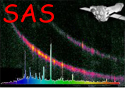XMM-Newton Science Analysis System

omdetect (omdetect-5.41) [xmmsas_20190531_1155-18.0.0]

## Extended Sources (non-mosaiced images)

Each extended source has a group of connected pixels associated with it, each one having a value greater than a minimum height above the corresponding background image pixel. These pixels can be seen by looking at the level image map (parameter levelimage) and are used to compute the source count-rate through the following steps:

• 1) The quantities the total raw pixel counts, total corrected raw pixel counts and the total raw background counts are initialised to zero.

Loop through each source pixel and

• a) Each image pixel is treated as a point-source and a 12 pixel (unbinned) aperture is centred on it. The total raw pixel counts is incremented by the image pixel value.

• b) The counts within the aperture are obtained and a coincidence-loss factor is computed.

• c) The pixel value is multiplied by the coincidence-loss correction and this value is added to the total corrected pixel counts.

• d) The total raw background counts is incremented by the value of the corresponding background-image pixel.

End of looping through each source pixel.

The raw count-rate is computed by subtracting the total raw background counts from the total raw pixel counts and dividing by the exposure counts. The quantity corrected background counts is computed by applying a coincidence-loss correction to the total raw background counts, using the mean background-counts per pixel and a 12 pixel (unbinned) aperture. This correction is generally negligible. The corrected count-rate is computed by subtracting the corrected background counts from the total corrected pixel counts and divied by the exposure.

XMM-Newton SOC/SSC -- 2019-06-02